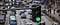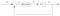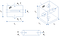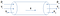Traffic control. Image via dymec.com

# Using an MPC to Control a System: Defining the System

Have you ever wondered how the cruise control in a car keeps its speed constant? How the pressure of a certain gas in an industrial container is controlled? Or even how your smatphone’s bightness adapts to the room’s light?

The automation of certain dynamic systems require application of control algorithms. A control algorithm is a loop that controls a system by decreasing the error between the desired variable, let’s say pressure inside the container, and a set-point for that same variable. The controller will then calculate a control input variable that changes the system so that the error in the next iteration is smaller. The control input could be an outlet valve that vents the excess gas for instance.Generic control loop. Image by author.

The figure above is the general schematics of a controller loop where our Open Loop System (OLS) may be a car, a gas container, a smartphone or whatever system you need to control. If we go with the gas container, a sensor reads the pressure in the kth iteration (P_k) that is going to be subracted to a reference value of pressure (P_R), our set-point. This provides an error for pressure in the kth iteration (ε_PK). The controller reads the error and computes a value for the control input variable (θ_K) that we can call a degree of openness of the valve that lets out excess pressure and best decreases the error of our control loop for the next iteration.

But how does a controller really work? What calculations does it do? Well, that depends on the type of controller we use. The most widely used is the PID controller for ease of use reasons. PID is very simple and easy to grasp and most often, the systems that need control, don’t demand for more complex technologies.

However, in this new series of publications, I’ll explore a more complex technology: the Model Predictive Control (MPC). This controller is different from PID as it requires a model of the real world system to make predictions.

How does it help?

Whereas a PID controls for only the next iteration, which makes much more prone to overshoot the target and wiggle around the set-point, modelling the system calculates predictions for several instants of time later. Thus, the MPC can calculate a more stable path of control and understand how the system will change several moments before it does.

Imagine a self driving car that is changing lanes. With a PID controller, at the end of the manoeuver it will be constantly zigzaging around the set-point because it doesn’t actually know that the second next point in time is in the same direction. It only cares to approach the set-point at that moment which causes the car to overshoot the straight line of the destination even if it is for a very small distance.

With the MPC controller, the car knows its destination is a straight line and the next iteration will be calculated not just to minimize the error for that iteration but also to minimize it for the following ones. This makes the car approach the line and stay in it.

Although the system I am going to model is a fairly simple one and could be easily contolled with PID, for practicing reasons, I’ll use an MPC.

## System modelling

Our system is a vessel for pre-heating a diluted flow stream of some aqueous solution with steam. Imagine we have an industry that produces excess steam and, to not let that energy go to waste, we use it to heat up the solution. In this situation, the solution is diluted enough so that we consider it to have water-like thermal characteristics.Cubic vessel with a cylindrical tube through the middle. On the left is a side profile of the vessel and on the right is the vessel in three dimensions. Bellow is the cylindrical tube. Image by author.

Figure above represents a schematics of our system. ṁ_s is the mass flowrate of the solution, ṁ_v is the mass flowrate of the steam, L_1 to L_3 are the spacial dimensions of the vessel and L_1 is also the length of the cillinder, D_c is the diameter of the cillinder, T_i and T_o is the inlet and outlet temperatures of the solution and finally, p_i and p_o are the inlet and outlet pressures of the steam.

The aqueous solution passes through a cubic vessel that we’ll consider perfectly agitated meaning that there are no temperature gradients in the solution and the steam passes through a cillinder inside the vessel. The steam is at 100 °C so that the heat provided to the solution is latent instead of sensible heat. We’ll consider 10 % condensation meaning only one tenth of the total available latent energy will be transfered to the solution. Also, the material of the cillinder has negligible heat resistance and all the heat lost by the steam will go to the solution. One last thing to have in mind is that the solution’s flowrate, unlike the steam’s flowrate, is constant.

## Temperature change

The modelization of our system will be of a Linear Time Invariant (LTI) shape. What does it mean? We’ll create a linear model where only the variables change with time. This is the simplest modelization. Others are Linear Time Variant (LTV) where variables as well as certain parameters change with time or even the most complex non-linear model where equations are just not linear at all.

Essentially, what we need to find is a equation that allows us to project the temperature of the solution over time since that is going to be the variable we want to control. So, we need to know the derivative of temperature with respect to (w.r.t.) time that we’ll call Ṫ (T dot) where the dot represents change over time and will be related to tempreature of outlet.

A simple energy balance can tell us the answer. Let’s understand how the energy transitions within the system. If we provide enough energy from steam, there should be an energy surplus that will work towards an increase in outlet temperature. That surplus is the accumulation meaning that steam provides enough energy for the inlet stream to gain an infinitesimal part more of energy than in the previous instant while inside the vessel. This will cause the outlet stream to come out with greater enthalpy and thus temperature.

Same line of thought could be taken for the other way around. Should the steam not provide enough energy for the solution stream to mantain its total energy at the outlet in the subsequent moment, there will be a reduction in total energy of the solution stream and thus, its temperature will fall.

Equation above shows what was discussed in the previous two paragraphs. The energy accumulated (or lost) (ΔH_acc) by the solution plus its energy variation between outlet (H_s,o) and inlet (H_s, i) at the previous instant must be equal to the energy provided by the water vapor (ΔH_v).

The energies are calculated with equations above. The energy entering the vessel with the inlet solution stream and exiting with the outlet stream are calculated multiplying the mass flowrate of solution with the specific heat capacity (Cp_s) of that same mass (we’ll use that of water since it is a diluted solution) and with its temperature.

The energy provided by the steam, since it is latent heat which doesn’t depend on temprature, is calculated multiplying its mass flowrate with the latent heat constant (λ) of water vapor. The 0.1 stands for the 10 % condensation. Let’s rearange the full equation to see what we get.

The three new constants are the residence time (τ) or the average time the solution stays inside the vessel, the volumetric flowrate (V dot) and the specific mass (ρ_v) of water vapor.

We have deduced our first equation. It will indicate how temperature changes in the various iterations our model will take. However, we still don’t know how the steam flowrate changes and this is of great importance since it is how we will control the solution’s temperature.

## Volumetric flowrate change

Now we need to understand the variation of the steam’s volumetric flowrate w.r.t. time. We’ll consider this flow to be non-viscous to avoid having to deal with friction on the sides of the cillinder. The variation in flowrate suposes either a variation in the velocity the steam travels along the cillinder or a variation in the mass of the steam. Can you guess where I am going with this? We’ll be dealing with Newton’s second law of motion but applied to fluid mechanics: the quantity of movement equation.

Looks like we have some vectors here. Let’s look at the vectors around the steam’s control volume. The control volume is the specific volume we are studying.Cylindrical tube in greater detail. Image by author.

The pressures are applied in the direction of the control volume. the velocities follow the direction of flow of steam and n_i and n_o are the normal vectors in both sides of the control volume (C.V.). The normal direction always points towards the outside of the volume. When dealing with vectors we need a referential that points us towards the positive direction which is defined by x.

Let’s define the forces applied to our C.V.:

p_o becomes negative because it points to the opposite way of our reference. Now let’s understand the following part:

This defines the quantity of movement that enters and exits the C.V. through a control area (C.A.). At any instant, if we consider that there is no accumulation of mass or considerable compressibility of steam inside the C.V., the quantity of movement coming in is the same as going out at any given instant. Because the normal vectors at both ends of the cillinder are opposite to each other, the internal product between the velocities and normal vectors will also be opposite:

Now we can unfold the integral and understand that its result is zero:

Now we need to understand how the quantity of movement inside the C.V. varies w.r.t. time with:

This term can be transformated into:

If we assume that there is no variation of mass within C.V. w.r.t. time, then:

By rearanging the variables we can find the acceleration of the steam’s flowrate (V dot dot). Finally we can put all terms together and obtain our final quantity of movement equation form.

Now, what does this mean physically? We can see that, as long as pressure at the inlet side is greater than at outlet side, the acceleration will be positive. This means the steam’s flowrate will keep increasing. If we lock pressure of outlet at atmosferic pressure (1 atm), only by creating some degree of vaccum will we be able to speed down the steam. This is important to understand so that we can create accurate boundaries when solving the problem.

## Wrapping up

As metioned above, this will be a small series where I’ll try to post one or two more publications about the MPC and its simulation.

In this post we talked about what are controllers meant for, how they work and examples were given. Then we stated a problem to solve with the MPC where we want to control the temperature of a solution and defined a model that fits the system in a LTI fashion with the following equations:

Next time we’ll continue building our model. In the mean time, take a look at this video from Steve Brunton that gives a good explanation on what are MPCs.

Thank you for reading and stay tuned!

## The Startup

Get smarter at building your thing. Join The Startup’s +787K followers.

## Sign up for Top 10 Stories

### By The Startup

Get smarter at building your thing. Subscribe to receive The Startup's top 10 most read stories — delivered straight into your inbox, once a week. Take a look.

By signing up, you will create a Medium account if you don’t already have one. Review our Privacy Policy for more information about our privacy practices.

Check your inbox
Medium sent you an email at to complete your subscription.

Written by

## Tiago Miguel

Chemical Engineer enthusiast in learning programming with python, data science and machine/deep learning skills

## The Startup

Get smarter at building your thing. Follow to join The Startup’s +8 million monthly readers & +787K followers.

Written by

## Tiago Miguel

Chemical Engineer enthusiast in learning programming with python, data science and machine/deep learning skills## The Startup

Get smarter at building your thing. Follow to join The Startup’s +8 million monthly readers & +787K followers.

## More From Medium

Medium is an open platform where 170 million readers come to find insightful and dynamic thinking. Here, expert and undiscovered voices alike dive into the heart of any topic and bring new ideas to the surface. Learn more

Follow the writers, publications, and topics that matter to you, and you’ll see them on your homepage and in your inbox. Explore

If you have a story to tell, knowledge to share, or a perspective to offer — welcome home. It’s easy and free to post your thinking on any topic. Write on Medium